# Analytical reasoning test-1

11)

In each of the following question, find the minimum number of triangles in the given figure.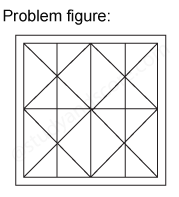12)

In each of the following question, find the minimum number of triangles in the given figure.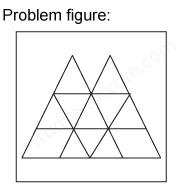13)

In each of the following question, find the minimum number of straight lines required to make the given figure.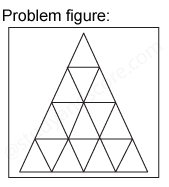14)

In each of the following question, find the minimum number of triangles in the given figure.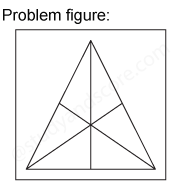15)

In each of the following question, find the minimum number of straight lines required to make the given figure.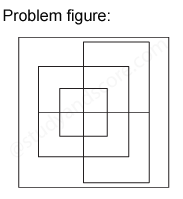--Share with your friends --